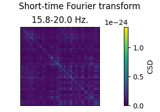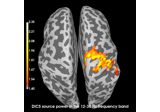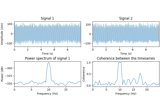# mne.time_frequency.CrossSpectralDensity#

class mne.time_frequency.CrossSpectralDensity(data, ch_names, frequencies, n_fft, tmin=None, tmax=None, projs=None)[source]#

Cross-spectral density.

Given a list of time series, the CSD matrix denotes for each pair of time series, the cross-spectral density. This matrix is symmetric and internally stored as a vector.

This object can store multiple CSD matrices: one for each frequency. Use `.get_data(freq)` to obtain an CSD matrix as an ndarray.

Parameters:
data`ndarray`, shape ((n_channels**2 + n_channels) // 2, n_frequencies)

For each frequency, the cross-spectral density matrix in vector format.

ch_names

List of string names for each channel.

frequencies

Frequency or frequencies for which the CSD matrix was calculated. When averaging across frequencies (see the `CrossSpectralDensity.mean()` function), this will be a list of lists that contains for each frequency bin, the frequencies that were averaged. Frequencies should always be sorted.

n_fft`int`

The number of FFT points or samples that have been used in the computation of this CSD.

tmin

Start of the time window for which CSD was calculated in seconds. Can be `None` (the default) to indicate no timing information is available.

tmax

End of the time window for which CSD was calculated in seconds. Can be `None` (the default) to indicate no timing information is available.

projs

List of projectors to apply to timeseries data when using this CSD object to compute a DICS beamformer. Defaults to `None`, which means no projectors will be applied.

Attributes:
`n_channels`

Number of time series defined in this CSD object.

Methods

 Subselect frequencies. Return number of frequencies. Return copy of the CrossSpectralDensity object. `get_data`([frequency, index, as_cov]) Get the CSD matrix for a given frequency as NumPy array. `mean`([fmin, fmax]) Calculate the mean CSD in the given frequency range(s). `pick_channels`(ch_names[, ordered]) Pick channels from this cross-spectral density matrix. `pick_frequency`([freq, index]) Get a CrossSpectralDensity object with only the given frequency. `plot`([info, mode, colorbar, cmap, n_cols, show]) Plot CSD matrices. `save`(fname, *[, overwrite, verbose]) Save the CSD to an HDF5 file. `sum`([fmin, fmax]) Calculate the sum CSD in the given frequency range(s).
__getitem__(sel)[source]#

Subselect frequencies.

Parameters:
sel`ndarray`

Array of frequency indices to subselect.

Returns:
csdinstance of `CrossSpectralDensity`

A new CSD instance with the subset of frequencies.

__len__()[source]#

Return number of frequencies.

Returns:
n_freqs`int`

The number of frequencies.

copy()[source]#

Return copy of the CrossSpectralDensity object.

Returns:
copyinstance of `CrossSpectralDensity`

A copy of the object.

get_data(frequency=None, index=None, as_cov=False)[source]#

Get the CSD matrix for a given frequency as NumPy array.

If there is only one matrix defined in the CSD object, calling this method without any parameters will return it. If multiple matrices are defined, use either the `frequency` or `index` parameter to select one.

Parameters:
frequency

Return the CSD matrix for a specific frequency. Only available when no averaging across frequencies has been done.

index

Return the CSD matrix for the frequency or frequency-bin with the given index.

as_cov`bool`

Whether to return the data as a numpy array (`False`, the default), or pack it in a `mne.Covariance` object (`True`).

New in version 0.20.

Returns:
csd`ndarray`, shape (n_channels, n_channels) | instance of `Covariance`

The CSD matrix corresponding to the requested frequency.

mean(fmin=None, fmax=None)[source]#

Calculate the mean CSD in the given frequency range(s).

Parameters:
fmin

Lower bound of the frequency range in Hertz. Defaults to the lowest frequency available. When a list of frequencies is given, these are used as the lower bounds (inclusive) of frequency bins and the mean is taken for each bin.

fmax

Upper bound of the frequency range in Hertz. Defaults to the highest frequency available. When a list of frequencies is given, these are used as the upper bounds (inclusive) of frequency bins and the mean is taken for each bin.

Returns:
csdinstance of `CrossSpectralDensity`

The CSD matrix, averaged across the given frequency range(s).

Examples using `mean`:Compute a cross-spectral density (CSD) matrix

Compute a cross-spectral density (CSD) matrixCompute source power using DICS beamformer

Compute source power using DICS beamformer
property n_channels#

Number of time series defined in this CSD object.

pick_channels(ch_names, ordered=False)[source]#

Pick channels from this cross-spectral density matrix.

Parameters:
ch_names

List of channels to keep. All other channels are dropped.

ordered`bool`

If True (default False), ensure that the order of the channels matches the order of `ch_names`.

Returns:
csdinstance of CrossSpectralDensity.

The modified cross-spectral density object.

Notes

Operates in-place.

New in version 0.20.0.

pick_frequency(freq=None, index=None)[source]#

Get a CrossSpectralDensity object with only the given frequency.

Parameters:
freq

Return the CSD matrix for a specific frequency. Only available when no averaging across frequencies has been done.

index

Return the CSD matrix for the frequency or frequency-bin with the given index.

Returns:
csdinstance of `CrossSpectralDensity`

A CSD object containing a single CSD matrix that corresponds to the requested frequency or frequency-bin.

plot(info=None, mode='csd', colorbar=True, cmap='viridis', n_cols=None, show=True)[source]#

Plot CSD matrices.

A sub-plot is created for each frequency. If an info object is passed to the function, different channel types are plotted in different figures.

Parameters:
info

The `mne.Info` object with information about the sensors and methods of measurement. Used to split the figure by channel-type, if provided. By default, the CSD matrix is plotted as a whole.

mode‘csd’ | ‘coh’

Whether to plot the cross-spectral density (‘csd’, the default), or the coherence (‘coh’) between the channels.

colorbar`bool`

Whether to show a colorbar. Defaults to `True`.

cmap

The matplotlib colormap to use. Defaults to None, which means the colormap will default to matplotlib’s default.

n_cols

CSD matrices are plotted in a grid. This parameter controls how many matrix to plot side by side before starting a new row. By default, a number will be chosen to make the grid as square as possible.

show`bool`

Whether to show the figure. Defaults to `True`.

Returns:
fig

The figures created by this function.

save(fname, *, overwrite=False, verbose=None)[source]#

Save the CSD to an HDF5 file.

Parameters:
fnamepath-like

The name of the file to save the CSD to. The extension `'.h5'` will be appended if the given filename doesn’t have it already.

overwrite`bool`

If True (default False), overwrite the destination file if it exists.

New in version 1.0.

verbose

Control verbosity of the logging output. If `None`, use the default verbosity level. See the logging documentation and `mne.verbose()` for details. Should only be passed as a keyword argument.

New in version 1.0.

`read_csd`

For reading CSD objects from a file.

sum(fmin=None, fmax=None)[source]#

Calculate the sum CSD in the given frequency range(s).

If the exact given frequencies are not available, the nearest frequencies will be chosen.

Parameters:
fmin

Lower bound of the frequency range in Hertz. Defaults to the lowest frequency available. When a list of frequencies is given, these are used as the lower bounds (inclusive) of frequency bins and the sum is taken for each bin.

fmax

Upper bound of the frequency range in Hertz. Defaults to the highest frequency available. When a list of frequencies is given, these are used as the upper bounds (inclusive) of frequency bins and the sum is taken for each bin.

Returns:
csdinstance of `CrossSpectralDensity`

The CSD matrix, summed across the given frequency range(s).

## Examples using `mne.time_frequency.CrossSpectralDensity`#DICS for power mapping

DICS for power mappingCompute a cross-spectral density (CSD) matrix

Compute a cross-spectral density (CSD) matrixCompute source level time-frequency timecourses using a DICS beamformer

Compute source level time-frequency timecourses using a DICS beamformerCompute source power using DICS beamformer

Compute source power using DICS beamformer